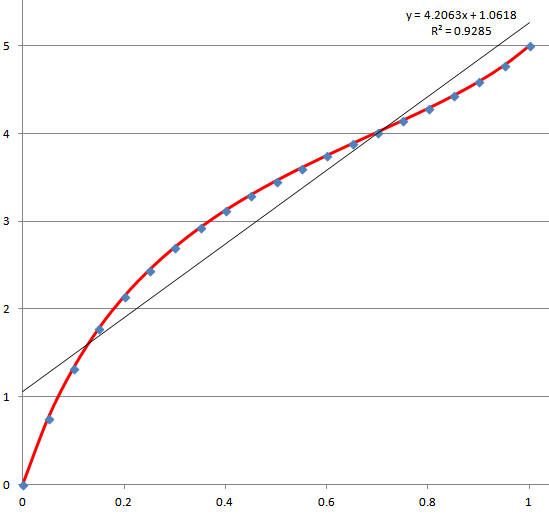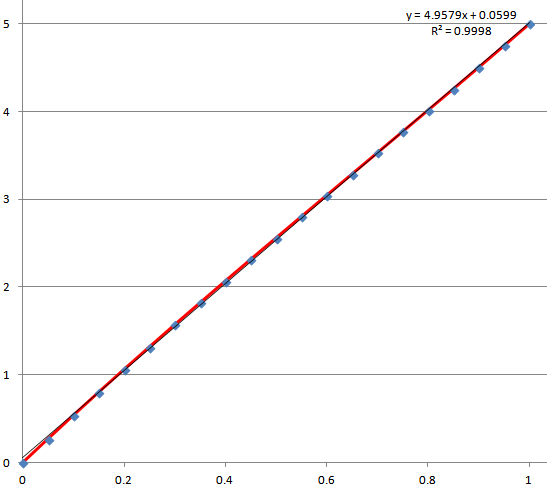## Voltage Level Shifting

14 posts / 0 new
Author
Message

Hi All,

I require a bit of analogue electronics level shifting guidance.

I am interfacing to an old circuit with a pot that is powered with -5V and +5V.  Therefore the output swing will be 10V (-5V to +5V).  I would like to feed the pot Vout voltage into a 5V ADC pin on a micro.

Is the preferred method to read and level shift this signal down to 0V to 5V, is to put this signal through a non-inverting op-amp, that is powered by -12V to +12V, and adjust the feedback resistors, so Vout is half Vin.

Look forward to any input, or if another circuit design is recommend.

Thanks,

Tuurbo46

```             ^ +5v
/ \
|R1 |
|   |
\  /
V
|
V   <R2>-----* U (analog MCUinput : huge impedance)

R1 == R2 edited : should be great w/r pot impedance -I do not know), and small w/r ADC input impedance (1E8 ohm)
U = ((R2*5) + (R1*V)) / (R1+R2) = 0.5* (5+V)```

Do you need a noninverting op -amp (at least 4 extra solders/wires) ?

Last Edited: Wed. Jun 6, 2018 - 11:25 AM

Thinking outside the square... replace your pot with a dual ganged one. On the new half, ground one end and connect  the other end to your +5 volts.

Ross McKenzie, Melbourne Australia

Don't you just need a 1:2 voltage divider with much higher impedance than the pot connected between VCC and the pot output?

It will swing 0 to +5V when the pot output swings -5V to +5V. Then, an RRIO opamp will buffer this signal and send it to the ADC.

Or, the divider is low impedance, so that it can connect directly to the ADC, but the pot output is buffered.

Perhaps old half will become useless.

Then, a pot with only one half, where -5v is replaced by zero volt (a wire/track to cut; two solders) would be enough.

Hi,

Thanks for your replies.

I cannot change or modify the pot as it is in an arm of an old machine.

So to conclude and as i understand with what El Tangas mentioned, the Op-Amp is required in series between the pot and micro for impedance buffering.  So my circuit idea is correct or not correct?

Thanks,

Tuurbo46

I assume you  can not simply connect the potentiometer between GND and +5?

What is the resistance of your potmeter?

Probably a simple divider with 2 resistors will do.

The sum of these resistors is preferably about 10x higher than the resistance of your potmeter.

Put the resistors in series between +5V and the wiper of the potmeter.

Connect the middle of those two resistors to the ADC input.

This will mean that if you only use only a single ADC channel the input impedance is very high.

You can test this by putting a small capacitor (pF, or low nF or so) to an analog input and then sample it's voltage repeatedly.

If you use however different ADC inputs, then the voltage of the internal sampling capacitor will get (dis) charged by the voltages on the input pins.

In this case the voltage levels on those other inputs wil cause a significant deviation in the voltage measured on the small capacitor mentioned before.

A fun experiment is to put a potentiometer to one ADC channel and only a capacitor to another ADC channel.

The ADC value on the capacitor wil (slowly) follow the ADC value from the potentiometer.

If multiple ADC channels are used you can "swamp" the sampling capacitor by using a bigger capacitor (100nF ?) parallel to the ADC input.

This will also have the benefit of filtering noise from the wiper of your potentiometer.

Doing magic with a USD 7 Logic Analyser: https://www.avrfreaks.net/comment/2421756#comment-2421756

Bunch of old projects with AVR's: http://www.hoevendesign.com

Which sample speed resolution etc.

Perhaps it would be easier to make a small -5 .. 5 V VCO, and let the AVR find the freq.

Last Edited: Wed. Jun 6, 2018 - 01:27 PM

funny I did not edit #8 !!!

Do you require linearity between the pot  position & the adc voltage?  If so , an opamp certainly works.

You can also "shift" the pot voltages by simply adding resistors (sort of a pullup/divider), though this might impact your linearity very slightly (the 10x factor mentioned helps to negate this, even 2x is not bad)

When in the dark remember-the future looks brighter than ever.   I look forward to being able to predict the future!

Tuurbo46 wrote:
So my circuit idea is correct or not correct?

Tuurbo46 wrote:
put this signal through a non-inverting op-amp, that is powered by -12V to +12V, and adjust the feedback resistors, so Vout is half Vin.

Well, not exactly, in my suggestion the opamp is not doing any amplifying, just being used as buffer for the pot or the voltage divider. But according to #7, maybe no buffering is even needed, you will just need to do some testing.

Hi,

Just to confirm my understanding of #7, Vout in the below diagram swings between 0-5V, when the pot is turned from end to end of its travel?

Thanks,

Tuurbo46Yes.

When the pot is all the way down, the voltage across the 5K/5K divider is +5 to - 5 V, so the mid-point is 0 V, with respect to Ground.

When the pot is all the way up, the voltage across the 5K/5K divider is +5 to +5 V, (no delta voltage), so no current flows through the divider, no voltage drop across either of those resistors, to the output is 5V, with respect to Ground.

JC

The loading of the r2/r3 divider causes a very slight nonlinearity, but at 5x it is very small.   If the  5k's were 200 ohms (1/5 k) the loading is much higher &  you can see the effect (which could actually be useful)At 5KWhen in the dark remember-the future looks brighter than ever.   I look forward to being able to predict the future!

Last Edited: Thu. Jun 7, 2018 - 01:06 PM